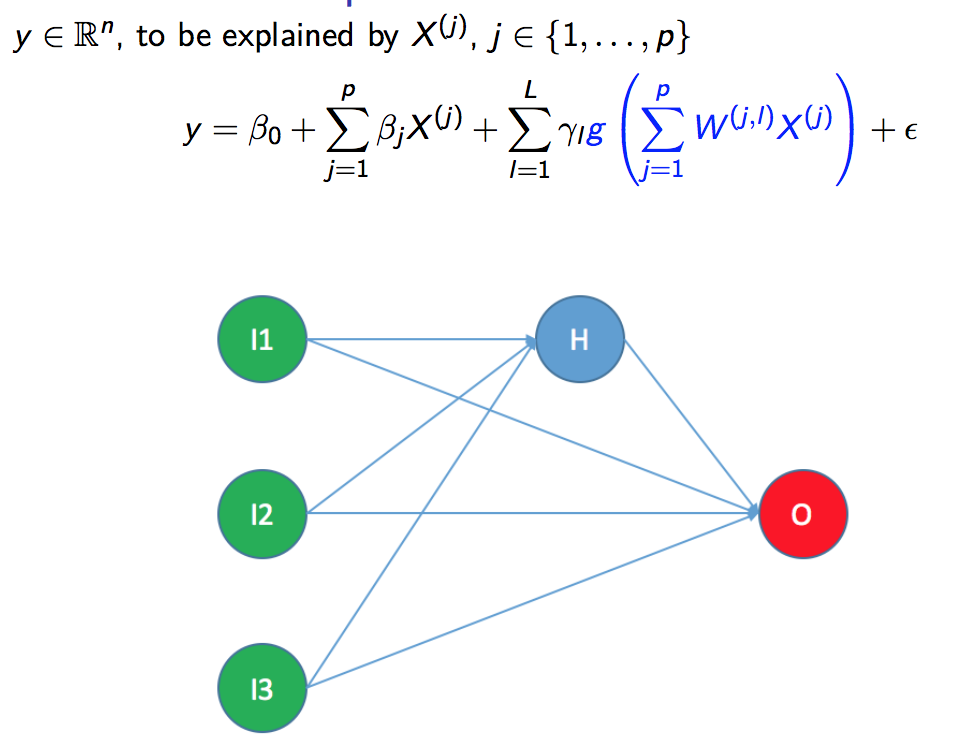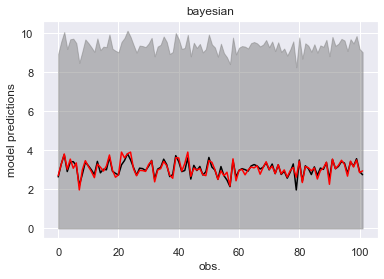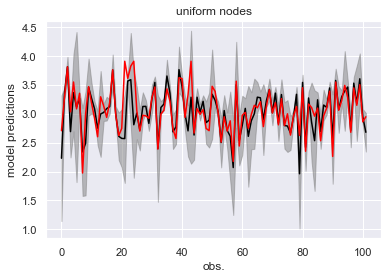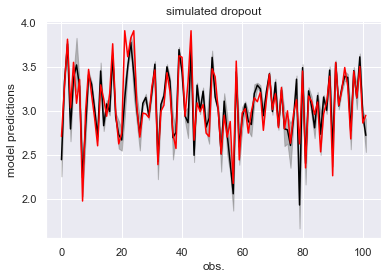There is always some uncertainty attached to Statistical/Machine Learning (ML) model’s predictions. In this post, we examine a few ways to obtain prediction intervals for nnetsauce’s ML models. As a reminder, every model in the nnetsauce is based on a component g(XW+b) where:

• X is a matrix containing some explanatory variables and optional clustering information (taking into account input data’s heterogeneity).
• W creates additional explanatory variables from X and is drawn from various random and quasirandom sequences.
• b is an optional bias parameter.
• g is an activation function such as the hyperbolic tangent or the sigmoid function.

If we consider again our example with tomatoes and apples, the y below (figure) contains our model’s decisions: either “observation is a tomato” or “observation is an apple”). The X’s are each fruit’s characteristics (color, shape, etc.).This figure presents the structure of a linear nnetsauce model but it could be anything including nonlinear models, as long as the model has methods fit and predict. There are many ways to obtain prediction intervals for nnetsauce models. Here, we present 3 ways for doing that:

• Using a bayesian linear model as a basis for our nnetsauce model
• Using a uniform distribution for W
• Using the randomness of dropout regularization technique on g(XW+b)

Here are the Python packages needed for our demo:

import nnetsauce as ns
import numpy as np
from sklearn import datasets
from sklearn import linear_model
from sklearn.model_selection import train_test_split


# Boston Housing data
X = boston.data
y = np.log(boston.target)

# split data in training/test set
X_train, X_test, y_train, y_test = train_test_split(X, y, test_size=0.2,
random_state=123)

##### Using a bayesian model as a basis for our nnetsauce model

Fitting a Bayesian Ridge regression model:

# base model for nnetsauce
regr = linear_model.BayesianRidge()

# create nnetsauce model based on the bayesian linear model
fit_obj = ns.CustomRegressor(obj=regr, n_hidden_features=10,
activation_name='relu', n_clusters=2)

# model fitting
fit_obj.fit(X_train, y_train)

# mean prediction and
# standard deviation of the predictions
mean_preds1, std_preds1 = fit_obj.predict(X_test, return_std=True)


The model’s prediction interval (using the mean prediction and the standard deviation of our predictions) is depicted below:Red line represents values of y_test; actual observed houses prices on unseen data (data not used for training the model). The black line represents model predictions on unseen data, and the shaded region is our prediction interval.

##### Using a uniform distribution for W

For doing that, we simulate the W in a nnetsauce CustomRegressor 100 times. With nodes_sim="uniform".

# number of model simulations
n = 100

# create nnetsauce models
fit_obj2 = [ns.CustomRegressor(obj=regr, n_hidden_features=10,
activation_name='relu', n_clusters=2,
nodes_sim="uniform",
seed=(i*1000 + 2)) for i in range(n)]

# model fitting
fit_obj2_fits = [fit_obj2[i].fit(X_train, y_train) for i in range(n)]

# models predictions
preds2 = np.asarray([fit_obj2_fits[i].predict(X_test) for i in range(n)]).T

# mean prediction
mean_preds2 = preds2.mean(axis=1)

# standard deviation of the predictions
std_preds2 = preds2.std(axis=1)



We obtain the following prediction interval:##### Using the dropout regularization technique on g(XW+b)

The dropout technique randomly drops some elements in the matrix g(XW+b). We can use its randomness to incorporate some uncertainty into our model:

# create nnetsauce models
fit_obj3 = [ns.CustomRegressor(obj=regr, n_hidden_features=10,
activation_name='relu', n_clusters=2,
nodes_sim="sobol", dropout=0.1,
seed=(i*1000 + 2)) for i in range(n)]

# models fitting
fit_obj3_fits = [fit_obj3[i].fit(X_train, y_train) for i in range(n)]

# model predictions
preds3 = np.asarray([fit_obj3_fits[i].predict(X_test) for i in range(n)]).T

# mean prediction
mean_preds3 = preds3.mean(axis=1)

# standard deviation of the predictions
std_preds3 = preds3.std(axis=1)


Using the dropout technique, we obtain:Using a uniform distribution for W seems to be the best way to obtain prediction intervals on this dataset and for this model. However, it’s worth mentioning that the base model (a bayesian linear model) is not calibrated. Calibrating the base model could lead to different results than those displayed here.

Note: I am currently looking for a gig. You can hire me on Malt or send me an email: thierry dot moudiki at pm dot me. I can do descriptive statistics, data preparation, feature engineering, model calibration, training and validation, and model outputs’ interpretation. I am fluent in Python, R, SQL, Microsoft Excel, Visual Basic (among others) and French. My résumé? Here!